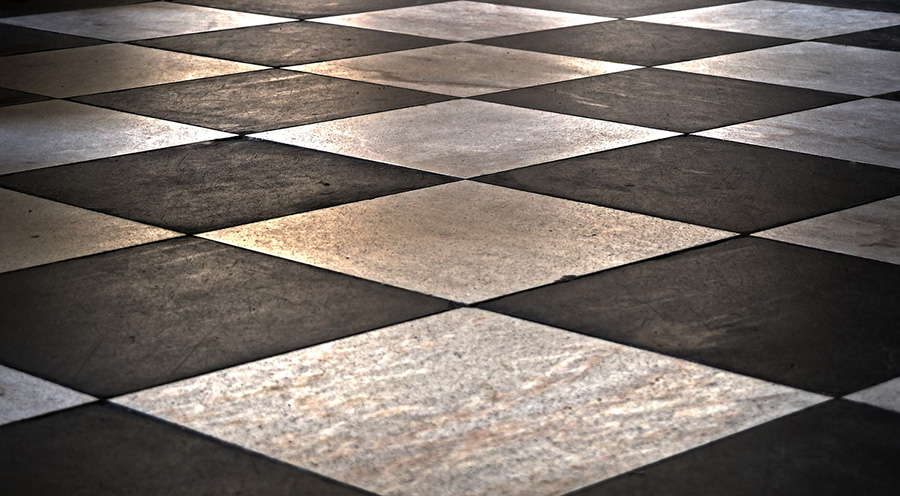# Tile Calculator

Estimate how many tiles you would need to cover an area with given dimensions. This tile calculator supports custom gap size and will also output the number of boxes of tiles and total cost given cost per box and tiles per box.

%
tiles/box
\$
Share calculator:

Embed this tool:
get code

## Calculating how many tiles you need

First, to calculate how many tiles you need to cover a given area you need to know the dimensions of the area as well as the dimensions of a single tile. The tile dimensions should be provided by the manufacturer, while the area dimensions can be measured using a long measuring tape or known from the building plan. Knowing these, our online tile calculator can calculate the area size and the area of a single tile.

From there, the basic formula to calculate the number of tiles required to cover an area is to divide the size of the area by the area of a single tile:

Number of tiles = area size / single tile area

This is, however, an idealized situation. Real-world tiles are not perfect, and they might have slight imperfections and discrepancies from the advertised dimensions. In order to compensate for those, gaps are left between tiles which are usually filled with grout, which is why they are often referred to as "grout lines". Usual values range from 1/16 to 1/2 an inch (2mm to 13mm) and the size varies depending on materials used and the intended design. For example, uniformly cut granite tiles allow for much smaller spacing between tiles, increasing the number of tiles needed.

Finally, you need to enter your expected percentage of wasted tiles. Waste usually occurs due to tiles being broken during handling and manipulation, but also due to the need to cut some of them in pieces in order to achieve the desired floor design / layout pattern. Some of these pieces cannot be used and need to be discarded. Allowing for between 5-10% waste is a good practice.

The calculator will also output the number of boxes and the total price of the tiles if you enter the box size (tiles per box) and the price per box. These two parameters are optional.## Does the calculation require a particular tile ordering?

No, the result of this tool should be applicable to any type of bond. You can use a linear grid with square/rectangular tiles, or angled squares, diamond shapes, etc. However, it is worth noting that some kinds of bonds like running bonds and herringbones usually result in a higher percentage of wasted bricks (~10%), so you might want to adjust for that by increasing the percentage of expected waste in the settings of our tool.

## Does the tile calculator support differently-shaped tiles?

While there are many different types of tile shapes, square or rectangular ones are most commonly used due to ease of installation and the low amount of waste with many layouts. Our calculator supports these two types, so if your design involves hexagonal, triangular, etc. shaped tiles you will need to do the math yourself.

#### Cite this calculator & page

If you'd like to cite this online calculator resource and information as provided on the page, you can use the following citation:
Georgiev G.Z., "Tile Calculator", [online] Available at: https://www.gigacalculator.com/calculators/tile-calculator.php URL [Accessed Date: 01 Apr, 2023].